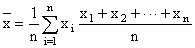Left Border Content - RF CafeAbout RF CafeCopyright: 1996 - 2024Webmaster:    Kirt Blattenberger,     BSEE - KB3UON RF Cafe began life in 1996 as "RF Tools" in an AOL screen name web space totaling 2 MB. Its primary purpose was to provide me with ready access to commonly needed formulas and reference material while performing my work as an RF system and circuit design engineer. The World Wide Web (Internet) was largely an unknown entity at the time and bandwidth was a scarce commodity. Dial-up modems blazed along at 14.4 kbps while typing up your telephone line, and a nice lady's voice announced "You've Got Mail" when a new message arrived... All trademarks, copyrights, patents, and other rights of ownership to images and text used on the RF Cafe website are hereby acknowledged. My Hobby Website: AirplanesAndRockets.comSub-Header - RF Cafe# Averages

As shown in the equations below, there are many different types of averages used in science and mathematics. The one that is probably the most familiar is the Arithmetic Mean.

Arithmetic Mean

To calculate the arithmetic mean with discrete random variables, add up all the terms, and then divide by the number of terms in the distribution. This type of average is commonly called the "average". The mean of a statistical distribution with a continuous random variable is the value of that random variable, formally denoted by the lowercase Greek letter mu (µ). The area under the probability density function curve to the left of µ is the same as the area under the curve to the right of µ (i.e., symmetry)., n is an integer

Arithmetic Mean Formula

Median

The median of a distribution with discrete random variables where the number of terms is odd then uses the value of the term in the middle. This is the value such that the number of terms having values greater than or equal to it is the same as the number of terms having values less than or equal to it. If the number of terms is even, then the median is the average of the two terms in the middle, such that the number of terms having values greater than or equal to it is the same as the number of terms having values less than or equal to it. The median of a distribution with a continuous random variable is the value m such that the probability is at least 0.5 (50%) that a randomly chosen point on the function will be less than or equal to m, and the probability is at least 0.5 that a randomly chosen point on the function will be greater than or equal to m.

Discrete Random Variable vs. Continuous Random Variable

Since the definitions of Median, Mode, and Range all depend on whether the variables are discrete or continuous, the difference is explained here for clarity. Discrete variables are a countable collection of values, e.g., the numbers 2.2, 5.9, 2.0, 3.4, 6.2, 4.5. There are exactly six values. The mean for them is, per the above formula, 24.2/6 = 4.0(3). The actual result on a calculator is 4.0333333...., but since the precision of all the numbers in the set is two significant figures, the result has to be the same. It has therefore been rounded down to 4.0.

A continuous variable refers to a mathematical function that takes on an infinite number of values between some lower and upper value. For instance, y = x2 [x=14] is the shape of a parabola and is defined for all values of x between 1 and 4. Therefore, y take on an infinite number of values between 1 and 16. Arriving at the answer requires using calculus to divide the integral of the range (x=14, which results in the value of 63) by the range of x (which is 4-1 = 3), to get 63/9 = 7. The precision of the range of x definition is one significant digit, so the answer is the same precision. In this case, even if the range of x was defined as x=1.00000 to x = 4.00000, the answer would still be exactly 7 (7.00000) because the ration 63/9 is exact.

Mode

The mode of a distribution is the value of the term that occurs the most often. Often a distribution will have more than one mode, especially if there are not many terms. This happens when two or more terms occur with equal frequency, and more often than any of the others. A distribution with two modes is called bimodal. A distribution with three modes is called trimodal. The mode of a distribution with a continuous random variable is the maximum value of the function. As with discrete distributions, there may be more than one mode.

Range

The range of a distribution with discrete random variables is the difference between the maximum value and the minimum value. For a distribution with a continuous random variable, the range is the difference between the two extreme points on the distribution curve, where the value of the function falls to zero. For any value outside the range of a distribution, the value of the function is equal to 0.

Weighted Arithmetic Mean, n is an integer

Weighted Arithmetic Mean

Geometric Mean, n is an integer

Geometric Mean

Harmonic Mean, n is an integer

Harmonic Mean

Root Mean Square (rms)n is an integer

Root Mean Square (rms)
Footer - RF CafeRight Border Content - RF CafePlease Support RF Cafe by purchasing my  ridiculously low−priced products, all of which I created. These Are Available for Free